Design of Joints

# Design of Joints - Notes | Study Transportation Engineering - Civil Engineering (CE)

 1 Crore+ students have signed up on EduRev. Have you?

Design of Joints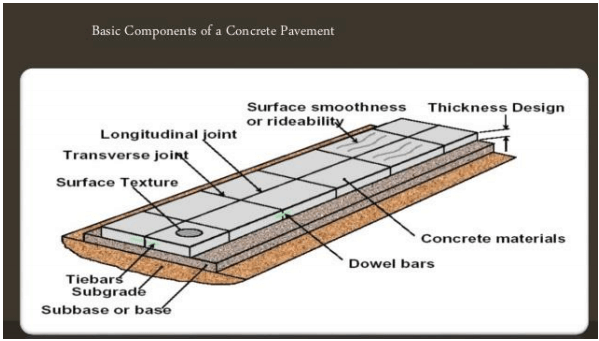Expansion joints
The purpose of the expansion joint is to allow the expansion of the pavement due to rise in temperature with respect to construction temperature. The design consideration are: 1.Provided along the longitudinal direction,
2.design involves finding the joint spacing for a given expansion joint thickness (say 2.5 cm specified by IRC) subjected to some maximum spacing (say 140 as per IRC)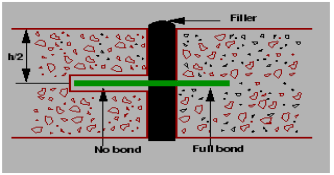Contraction joints
The purpose of the contraction joint is to allow the contraction of the slab due to fall in slab temperature below the construction temperature. The design considerations are:
The movement is restricted by the sub-grade friction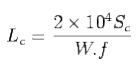where, Sis the allowable stress in tension in cement concrete and is taken as 0.8 kg/cm2 , is the unit weight of the concrete which can be taken as 2400 kg/cm3 and f is the coefficient of sub-grade friction which can be taken as 1.5.
Steel reinforcements can be use, however with a maximum spacing of 4.5 m as per IRC.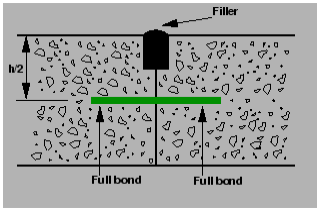Figure: Contraction joint

Dowel bars
The purpose of the dowel bar is to effectively transfer the load between two concrete slabs and to keep the two slabs in same height. The dowel bars are provided in the direction of the traffic (longitudinal). The design considerations are:
 Mild steel rounded bars,
 bonded on one side and free on other side

Bradbury's analysis gives load transfer capacity of single dowel bar in shear, bending and bearing as follows: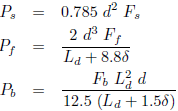where, P is the load transfer capacity of a single dowel bar in shear s, bending f and bearing b, d is the diameter of the bar in cm, Ld is the length of the embedment of dowel bar in cm, is the joint width in cm, Fs; Ff; Fb are the permissible stress in shear, bending and bearing for the dowel bar in kg/cm2 .

Design procedure
Step 1 Find the length of the dowel bar embedded in slab Ld by equating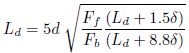Step 2 Find the load transfer capacities Ps, Pf , and Pb of single dowel bar with the Ld.
Step 3 Assume load capacity of dowel bar is 40 percent wheel load, _nd the load capacity factor f as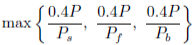Step 4 Spacing of the dowel bars.
Effective distance upto which effective load transfer take place is given by 1:8 l, where l is the radius of relative stiffness.
Assume a linear variation of capacity factor of 1.0 under load to 0 at 1:8 l.
Assume a dowel spacing and find the capacity factor of the above spacing.
Actual capacity factor should be greater than the required capacity factor.
If not, do one more iteration with new spacing.

Tie bars
In contrast to dowel bars, tie bars are not load transfer devices, but serve as a means to tie two slabs. Hence tie bars must be deformed or hooked and must be anchored into the concrete to function properly. They are smaller than dowel bars and placed at large intervals. They are provided across longitudinal joints.
Step 1 Diameter and spacing: The diameter and the spacing is found out by equating the total sub-grade friction to the total tensile stress for a unit length (one meter). Hence the area of steel per one meter in
cm2 is given by: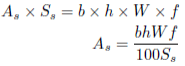where, b is the width of the pavement panel in m, h is the depth of the pavement in cm, W is the unit weight of the concrete (assume 2400 kg=cm2), f is the coefficient of friction (assume 1:5), and Ss is the allowable working tensile stress in steel (assume 1750 kg=cm2). Assume 0:8 to 1:5 cm _ bars for the design.
Step 2 Length of the tie bar: Length of the tie bar is twice the length needed to develop bond stress equal to the working tensile stress and is given by: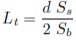Where, d is the diameter of the bar, Ss is the allowable tensile stress in kg=cm, and Sb is the allowable bond stress and can be assumed for plain and deformed bars respectively as 17:5 and 24:6 kg=cm2 .

Factors Affecting Pavment  Design
Traffic is the most important factor in the pavement design. The key factors include contact pressure, wheel load, axle configuration, moving loads, load, and load repetitions. Contact pressure
The tyre pressure is an important factor, as it determine the contact area and the contact pressure between the wheel and the pavement surface. Even though the shape of the contact area is elliptical, for sake of simplicity in analysis, a circular area is often considered.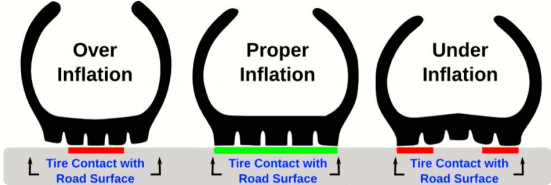The next important factor is the wheel load which determines the depth of the pavement required to ensure that the subgrade soil is not failed. Wheel configuration affect the stress distribution and deflection within a pavemnet. Many commercial vehicles have dual rear wheels which ensure that the contact pressure is within the limits. The normal practice is to convert dual wheel into an equivalent single wheel load so that the analysis is made simpler.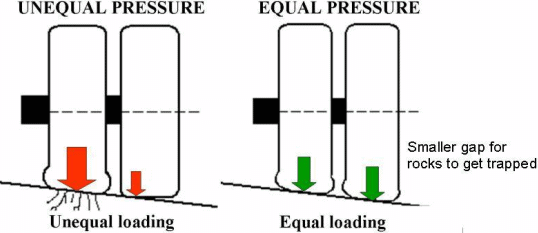Axle configuration The load carrying capacity of the commercial vehicle is further enhanced by the introduction of multiple axles.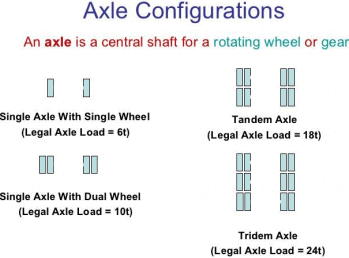Moving loads The damage to the pavement is much higher if the vehicle is moving at creep speed. Many studies show that when the speed is increased from 2 km/hr to 24 km/hr, the stresses and deflection reduced by 40 per cent.
Repetition of Loads The influence of traffic on pavement not only depend on the magnitude of the wheel load, but also on the frequency of the load applications. Each load application causes some deformation and the total deformation is the summation of all these. Although the pavement deformation due to single axle load is very small, the cummulative effect of number of load repetition is significant. Therefore, modern design is based on total number of standard axle load (usually 80 kN single axle).

Material characterization
The following material properties should be specified for both flexible and rigid pavements.
• When pavements are considered as linear elastic, the elastic moduli and poisson ratio of subgrade and each component layer must be specified.
• If the elastic modulus of a material varies with the time of loading, then the resilient modulus, which is elastic modulus under repeated loads, must be selected in accordance with a load duration corresponding to the vehicle speed.
• When a material is considered non-linear elastic, the constitutive equation relating the resilient modulus to the state of the stress must be provided.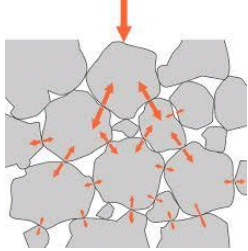Environmental factors
Environmental factors affect the performance of the pavement materials and cause various damages. Environ- mental factors that affect pavement are of two types, temperature and precipitation and they are discussed below:

Temperature
The effect of temperature on asphalt pavements is different from that of concrete pavements. Temperature affects the resilient modulus of asphlat layers, while it induces curling of concrete slab. In rigid pavements, due to difference in temperatures of top and bottom of slab, temperature stresses or frictional stresses are developed. While in flexible pavement, dynamic modulus of asphaltic concrete varies with temperature. Frost heave causes differential settlements and pavement roughness. The most detrimental effect of frost penetration occurs during the spring break up period when the ice melts and subgrade is a saturated condition.

Precipitation
The precipitation from rain and snow affects the quantity of surface water infiltrating into the subgrade and the depth of ground water table. Poor drainage may bring lack of shear strength, pumping, loss of support, etc.
To carry maximum load with in the specified limit and to carry greater load, dual wheel, or dual tandem assembly is often used. Equivalent single wheel load (ESWL) is the single wheel load having the same contact pressure, which produces same value of maximum stress, deflection, tensile stress or contact pressure at the desired depth. The procedure of finding the ESWL for equal stress criteria is provided below. This is a semi-rational method, known as Boyd and Foster method, based on the following assumptions:
• equalancy concept is based on equal stress;
• contact area is circular;
• influence angle is 45o ; and
• soil medium is elastic, homogeneous, and isotropic half The ESWL is given by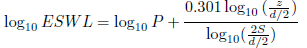Where P is the wheel load, S is the Center to center distance between the two wheels d is the clear distance between two wheels, and z is the desired depth.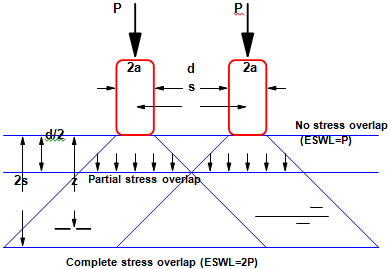The document Design of Joints - Notes | Study Transportation Engineering - Civil Engineering (CE) is a part of the Civil Engineering (CE) Course Transportation Engineering.
All you need of Civil Engineering (CE) at this link: Civil Engineering (CE)

## Transportation Engineering

22 videos|68 docs|58 tests

## Transportation Engineering

22 videos|68 docs|58 tests

Track your progress, build streaks, highlight & save important lessons and more!

,

,

,

,

,

,

,

,

,

,

,

,

,

,

,

,

,

,

,

,

,

;